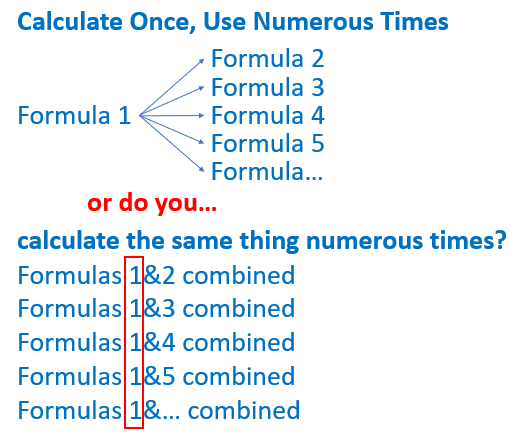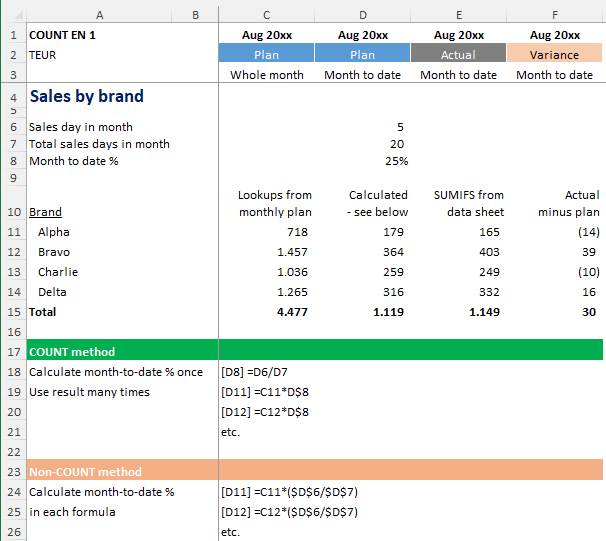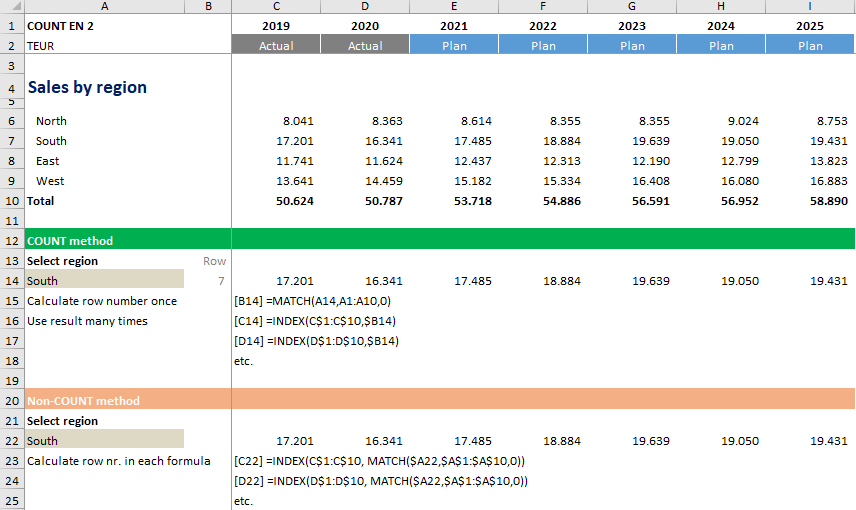# Do you COUNT?

COUNT stands for ‘Calculate Once, Use Numerous Times’. That means if you need to use a value multiple times, you should calculate it just the once and then link back to that value in all subsequent calculations. This can make your calculations shorter, easier to understand and faster.

Here you can see the logic. Using the COUNT method is simply more efficient.Here are two examples to show how this works...

Example 1: Actual vs plan sales, month to dateIn this example I want to compare the actual month-to-date sales with the planned month-to-date sales for each brand. For the planned sales I only have figures for the whole month and need to multiply these with the percentage of sales days so far (the month-to-date %).

• COUNT method: The month-to-date % is the same for all brands so I calculate the figure once and use the result for each brand.
• Non-COUNT method: I calculate the month-to-date % multiple times, once for each brand.

The COUNT method is more efficient than calculating the percentage again and again for each and every brand and it speeds up calculations. This is particularly important for large or complex spreadsheets that can take a while to calculate.

Example 2: Sales by year for a selected regionThis example uses INDEX and MATCH. Here we want to retrieve the annual sales values for a selected region.

• COUNT method: The data for the selected region is always in the same row, so I only need to calculate this row number once (with MATCH) for use in all INDEX formulas.
• Non-COUNT method: The row number is calculated again and again, in each INDEX formula.

Using the COUNT method, the formulas are much shorter than the non-COUNT method, and so are easier to understand and also faster.

This is just one of the many tips I explain in my book Avoid Excel Horror Stories. Read more here or buy it on Amazon.